Next: Gravity Waves at an Up: Waves in Incompressible Fluids Previous: Ship Wakes

# Gravity Waves in a Flowing Fluid

Consider a gravity wave traveling through a fluid that is flowing horizontally at the uniform velocity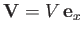. Let us write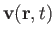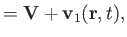(11.84)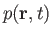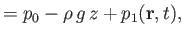(11.85)

where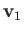and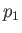are the small velocity and pressure perturbations, respectively, due to the wave. To first order in small quantities, the fluid equations of motion, (11.1) and (11.2), reduce to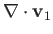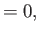(11.86)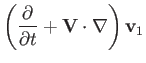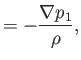(11.87)

respectively. We can also define the displacement,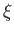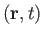, of a fluid particle due to the passage of the wave, as seen in a frame co-moving with the fluid, as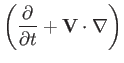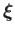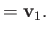(11.88)

The curl of Equation (11.87) implies that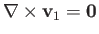. Hence, we can write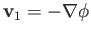, and Equation (11.87) yields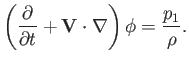(11.89)

Finally, Equation (11.86) gives(11.90)

The most general traveling wave solution to Equation (11.90), with wave vector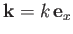, and angular frequency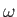, is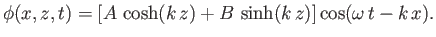(11.91)

It follows from Equation (11.89) that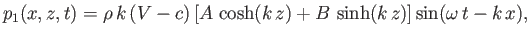(11.92)

and from Equation (11.88) that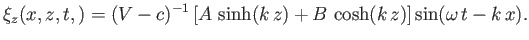(11.93)

Here,is the phase velocity of the wave.Next: Gravity Waves at an Up: Waves in Incompressible Fluids Previous: Ship Wakes
Richard Fitzpatrick 2016-03-31